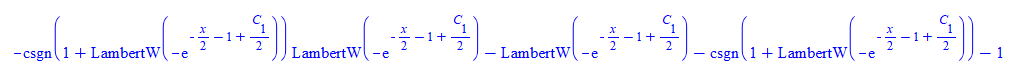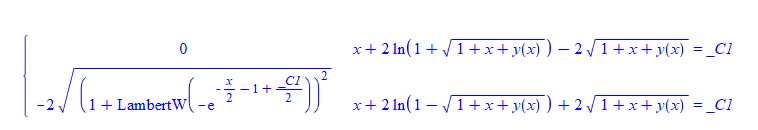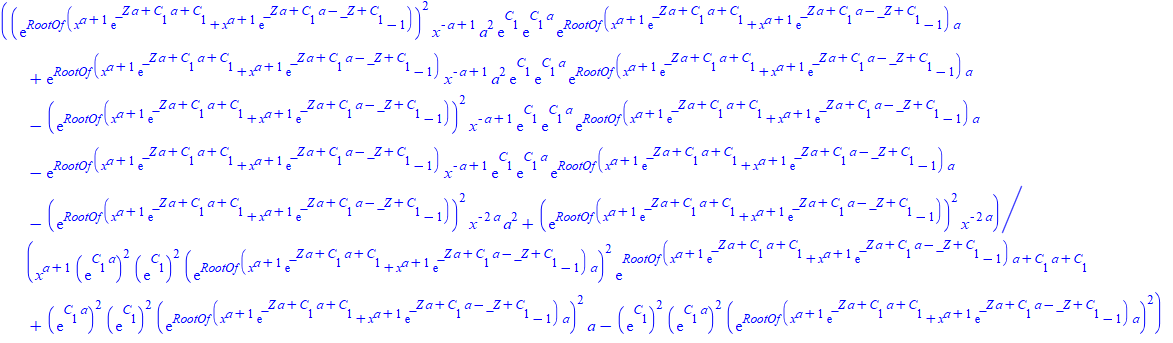Question:another problem with odetest. Verification depends on what constant of integration is called

Question:another problem with odetest. Verification depends on what constant of integration is called

Maple 2020

I think I've seen such a problem before but thought it was fixed.

Here is an example, where depending on what one calls the constant of integration, maple can or not, verify the solution of the ode.

This should not happen.

When calling the constant of integration C or _C Maple does not verify the solution. Only when using _C1 it does.

This is a problem, since I do not use _C1 or _C2  in my solution (so not to conflict with Maple's), I use C and C.

Is there a workaround for this?

Example 1

ode:=diff(z(x),x)+z(x)*cos(x)=z(x)^n*sin(2*x);
mysol:=z(x) = 1/((_C1*exp(sin(x)*(n - 1))*n - _C1*exp(sin(x)*(n - 1)) + 2*sin(x)*n - 2*sin(x) + 2)/(n - 1))^(1/(n - 1));
odetest(mysol,ode);

0

Now I just changed _C1 to C

restart;
ode:=diff(z(x),x)+z(x)*cos(x)=z(x)^n*sin(2*x);
mysol:=z(x) = 1/(( C*exp(sin(x)*(n - 1))*n - C*exp(sin(x)*(n - 1)) + 2*sin(x)*n - 2*sin(x) + 2)/(n - 1))^(1/(n - 1));
odetest(mysol,ode);

and I get 2 pages full of stuff thrown at the screen. Same when using C as constant of integration.

I could change my C and C to _C1 and _C2 each time just before calling odetest as workaround.

But would like to ask if this is supposed to happen and why it happens. I depend on odetest alot, as I use it to tell me if my solution is correct or not and I do not want false negatives.

I have found additional ones. May be these will help locate the problem

Example 2

restart;
ode:=diff(y(x),x) = (1+x+y(x))^(1/2);
mysol1:=y(x) = LambertW(-exp(-x/2 - 1 + C/2))^2 + 2*LambertW(-exp(-x/2 - 1 + C/2)) - x:
odetest(mysol1,ode);

givesrestart;
ode:=diff(y(x),x) = (1+x+y(x))^(1/2):
mysol2:=y(x) = LambertW(-exp(-x/2 - 1 + _C1/2))^2 + 2*LambertW(-exp(-x/2 - 1 + _C1/2)) - x:
odetest(mysol2,ode);Example 3

restart;
ode:=diff(y(x),x) = f(x)*y(x)+g(x)*y(x)^k:
mysol1:=y(x) = (-exp(-(k - 1)*Int(f(x), x))*(int(g(x)*exp((k - 1)*Int(f(x), x)), x)*k - int(g(x)*exp((k - 1)*Int(f(x), x)), x) - C))^(-1/(k - 1)):
odetest(mysol1,ode);

#long output is given

Changing C to _C1 gives 0 right away

restart;
ode:=diff(y(x),x) = f(x)*y(x)+g(x)*y(x)^k:
mysol2:=y(x) = (-exp(-(k - 1)*Int(f(x), x))*(int(g(x)*exp((k - 1)*Int(f(x), x)), x)*k - int(g(x)*exp((k - 1)*Int(f(x), x)), x) - _C1))^(-1/(k - 1)):
odetest(mysol2,ode);

0

Example 4

restart;
ode:=3*y(x)*diff(y(x),x)+5*cot(x)*cot(y(x))*cos(y(x))^2 = 0:
mysol1:=y(x) = RootOf(10*ln(sin(x))*cos(_Z)^2 - 3*cos(_Z)*sin(_Z) + 30*C*cos(_Z)^2 + 3*_Z):
odetest(mysol1,ode);

Gives pages long output. Changing C to _C1 gives zero

restart;
ode:=3*y(x)*diff(y(x),x)+5*cot(x)*cot(y(x))*cos(y(x))^2 = 0:
mysol2:=y(x) = RootOf(10*ln(sin(x))*cos(_Z)^2 - 3*cos(_Z)*sin(_Z) + 30*_C1*cos(_Z)^2 + 3*_Z):
odetest(mysol2,ode);

0

Example 5

restart;
ode:=x*(x-a*y(x))*diff(y(x),x) = y(x)*(y(x)-a*x);
mysol1:=y(x) = exp((-a + 1)*RootOf(x*exp(ln(x)*a + _Z*a + C*a + C) + exp(ln(x)*a + _Z*a + C*a - _Z + C)*x - 1) - ln(x)*a - C*(a + 1)):
odetest(mysol1,ode);

givesChanging C to _C1 gives zero

restart;
ode:=x*(x-a*y(x))*diff(y(x),x) = y(x)*(y(x)-a*x);
mysol2:=y(x) = exp((-a + 1)*RootOf(x*exp(ln(x)*a + _Z*a + _C1*a + _C1) + exp(ln(x)*a + _Z*a + _C1*a - _Z + _C1)*x - 1) - ln(x)*a - _C1*(a + 1)):
odetest(mysol2,ode);

0

Example 6

restart;
ode:=x*diff(y(x),x)^2-a*y(x)*diff(y(x),x)+b = 0:
mysol1:=y(x) = x*RootOf(2*x*_Z^2*a - 2*C*_Z^((2*a - 1)/(a - 1))*a - x*_Z^2 + C*_Z^((2*a - 1)/(a - 1)) - b)/a + b/(RootOf(2*x*_Z^2*a - 2*C*_Z^((2*a - 1)/(a - 1))*a - x*_Z^2 + C*_Z^((2*a - 1)/(a - 1)) - b)*a):
odetest(mysol1,ode);

gives many pages of output. Changing C to _C1 gives zero

restart;
ode:=x*diff(y(x),x)^2-a*y(x)*diff(y(x),x)+b = 0:
mysol2:=y(x) = x*RootOf(2*x*_Z^2*a - 2*_C1*_Z^((2*a - 1)/(a - 1))*a - x*_Z^2 + _C1*_Z^((2*a - 1)/(a - 1)) - b)/a + b/(RootOf(2*x*_Z^2*a - 2*_C1*_Z^((2*a - 1)/(a - 1))*a - x*_Z^2 + _C1*_Z^((2*a - 1)/(a - 1)) - b)*a):
odetest(mysol2,ode);

0

and more. I think the above should hopefully help locate the issue.

Maple 2020.1 with Physics 708﻿